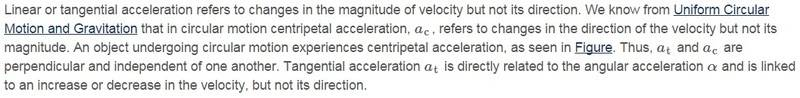# Introductory Rotational Motion Question

• Jazz
In summary, the paragraph explains that acceleration is a vector quantity, which has both magnitude and direction. Linear acceleration is a change in magnitude, while centripetal acceleration is a change in direction.f

#### Jazz

I'm beginning the chapter of Rotational Motion and Angular Momentum and it says the following which got me confused:When I was introduced acceleration at the beginning, it was stated that an acceleration ##a = \frac{\Delta v}{\Delta t}## can be a change either in speed's magnitude or direction; or both. In other words, in any case I would be dealing with an acceleration ##a##.

Does it mean that this is not true?
Do I need to be more specific?
If linear acceleration ##a_t## is a change in the speed's magnitude and ##a_c## a change in its direction, how is the change in speed's magnitude and direction at the same time called? Just acceleration?

Thanks!

Velocity is a vector quantity, has both magnitude and direction. ## \vec v = v \vec {e}## where v is the magnitude of the velocity (called 'speed') and ##\vec e ## is an unit vector in the direction of the velocity.

Acceleration is also vector, the time derivative of the velocity: ##\vec a = \frac {d \vec v}{dt}##. Applying product rule ## \vec a = \frac {d \vec v}{dt} = \frac {d (v \vec e)}{dt}= \frac{dv}{dt} \vec e + v \frac {d \vec e}{dt}##. The first term is acceleration in the original direction: linear acceleration at. The second term is the centripetal acceleration, corresponding to the change of the direction.

Velocity is a vector quantity, has both magnitude and direction. ## \vec v = v \vec {e}## where v is the magnitude of the velocity (called 'speed') and ##\vec e ## is an unit vector in the direction of the velocity.

Acceleration is also vector, the time derivative of the velocity: ##\vec a = \frac {d \vec v}{dt}##. Applying product rule ## \vec a = \frac {d \vec v}{dt} = \frac {d (v \vec e)}{dt}= \frac{dv}{dt} \vec e + v \frac {d \vec e}{dt}##. The first term is acceleration in the original direction: linear acceleration at. The second term is the centripetal acceleration, corresponding to the change of the direction.

Aah, I see that the statement of the paragraph above does not contradict what I learned about acceleration, as I was thinking.

Now it's much clearer.

Thank you :)